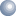## What is Power Factor?

Power factor is the ratio between the kW and the kVA drawn by an electrical load where the kW is the actual load power and the kVA is the apparent load power.

Simply, it is a measure of how efficiently the load current is being converted into useful work output and more particularly is a good indicator of the effect of the load current on the efficiency of the supply system.

Consider this:

When you buy fuel for a vehicle, the manufacturer makes in it in litres, the pump dispenses it in litres and you pay for it in litres. £/litre – simple!

When you buy potatoes, the supplier bags them in kilos the shop sells them in kilos and you pay for them in kilos. £/kg – simple!

When you buy electricity, the “manufacturer” (electricity generator) makes kVA (kilo volt amperes) and you pay for it in kWh (kilowatt hours) or maybe on your bill (Units) – not so simple!

Maybe we all should have kVA meters to make life simple.

So what is the kilowatt hour (or unit) we get on our bills? Simply, 1000 watts of electricity being used for 1 hour.

Example: 10 x 100 watt lamps x 1 hour=1000 watts/hr divided by 1000=1kWh – simple!

Now here comes the problem: In an alternating current (AC) electrical supply, a mysterious thing called “Power Factor” comes into play. Power Factor is simply the measure of the efficiency of the power being used, so, a power factor of 1 would mean 100% of the supply is being used efficiently. A power factor of 0.5 means the use of the power is very inefficient or wasteful.

So what causes Power Factor to change? In the real world of industry and commerce, a power factor of 1 is not obtainable because equipment such as electric motors, welding sets, fluorescent and high bay lighting create what is called an “inductive load” which in turn causes the amps in the supply to lag the volts. The resulting lag is called Power Factor.

For a 3 phase power supply: kVA, which the electricity generator makes=Line Volts x Amps x 1.73÷ 1000. This is converted to kilowatts kW by the formula: Line Volts x Amps x 1.73 ÷ 1000 x Power Factor=kW (V x A x 1.73÷ 1000 x pf) or kVA x pf=kW (N.B. 1.73 is the square root of 3) so as the power factor worsens from say 0.98 to 0.5, the generator has to supply more kVA for each kW you are using.

For example, a large electric motor will typically have a Power Factor of about 0.85 at full load. If we have a hypothetical electric motor rated at 100kW, then ignoring the inherent inefficiency of the motor, when running at full load the electricity supplier would have to supply 100÷0.85=118kVA to provide the 100kW to run the motor.

Or put the other way they would be supplying 18% more electricity than they are charging you for. If the same motor was operating “off load” at say 50kW or being used on a cyclic duty then the power factor may go as low as 0.5.

In this case the supplier would have to supply “double” the kVA to match the 50kW duty point. (50÷0.5=100kVA)

How this power is wasted can be shown graphically since in 3 phase power supplies "power" can be represented and measured as a triangle. ACTIVE Power is the base line and is the “real” usable power measured and paid for in kW. REACTIVE power is the vertical or that part of the supply which causes the inductive load. The reactive power in is measured in kVAr (kilo volt-amperes reactive). APPARENT Power is the hypotenuse. This is the component the electricity generator must supply and it is the resultant of the other two components, measured in kVA. Mathematically the power can be calculated by pythagoras or trigonometry whereby Power Factor is expressed as COS phi Ø (The angle between Apparent Power and Active power)

But we want a simple explanation so consider a barge being pulled by a horse:Since the horse cannot walk on water its pulling effort is reduced by the “angle” of the tow rope.

If the horse could walk on water then the angle Phi Ø would be zero and COSINE Ø=1. Meaning all the horse power is being used to pull the load.

However the relative position of the horse influences the power. As the horse gets closer to the barge, angle Ø1 increases and power is wasted, but, as the horse is positioned further away, then angle Ø2 gets closer to zero and less power is wasted

So, by improving Power Factor (reducing the angle), the reactive power component is reduced

What does it do to my electricity bill?

As stated above in a 3 phase power supply, kW consumed is 3 phase VOLTS x AMPS x 1.73 x Power Factor. The Electricity Company supply you VOLTS x AMPS and they have to supply extra to make up for the loss caused by poor Power Factor.

When the power factor falls below a set figure, the electricity supply companies charge a premium on the kW being consumed, or, charge for the whole supply as kVA by adding reactive power charges (kVar) to the bill.

How does Power Factor Correction work?

By installing suitably sized switched capacitors into the power distribution circuit, the Power Factor is improved and the value becomes nearer to 1 thus minimising wasted energy, improving the efficiency of a plant, liberating more kW from the available supply and saving you money!

The purchase cost of the installation is usually repaid in less than 1 years electricity savings.This document maintained by ajmenergy@btinternet.com.
.